# 全职业-剑鬼CP动图、护石符文提升与选择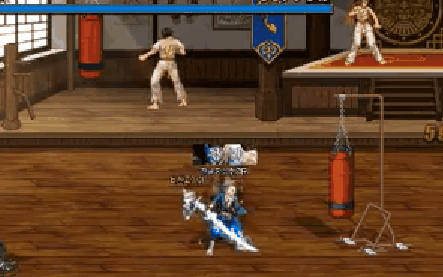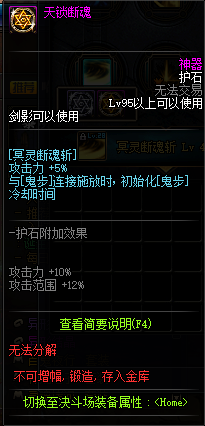100级工作服情况下，断魂斩等级可以达到 31(等级)+6（特色）=37级

X% CD 40S

X%*1.15*1.095=1.25925X% CD 40S

1.25925X%/X%-1=25.9%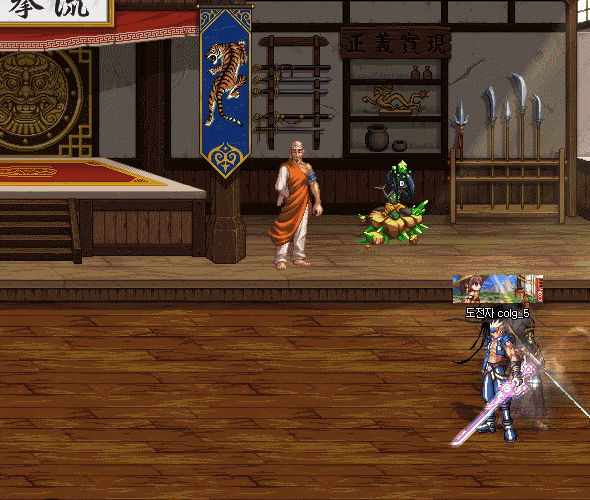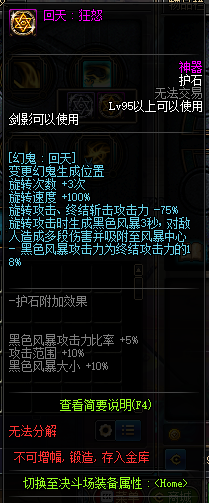100级工作服情况下，回天等级可以达到 33(等级)+6（特色）=39级

X%+0.67*X%=1.67X% CD 17S

(0.67为回旋相对于终结部分伤害占比）

0.25*X%+0.25*0.67*X%*4+0.25*4*X%*0.18*1.05*6=2.064X% CD 17S

（6为3秒风暴攻击次数）

2.064X%/1.67X%-1=24%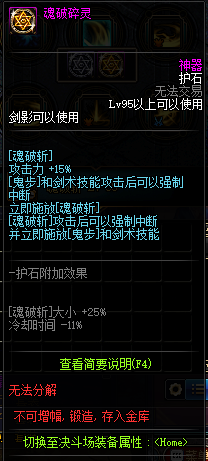100级工作服情况下，魂破斩等级可以达到36(等级)+6（特色）=42级

X% CD 10S

X%*1.15=1.15X% CD 9 S

1.15X%/X%-1=15%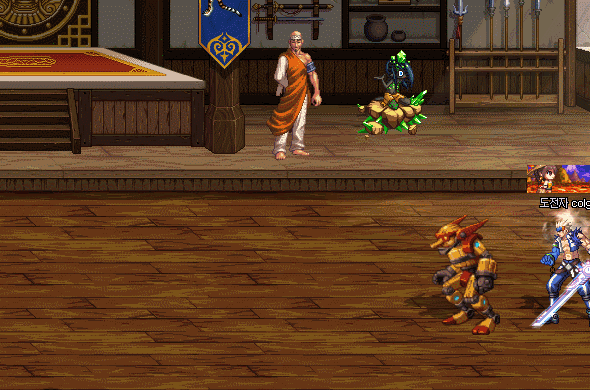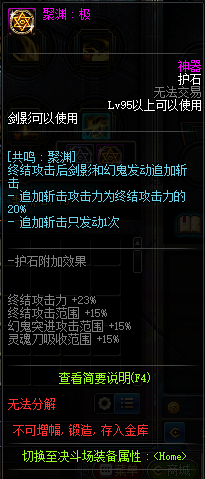100级工作服情况下，聚渊等级可以达到18级

X%+0.25*X%+1.25*X%=2.5X% CD 44.5S

（0.25为幻鬼移动伤害相对于终结攻击力伤害部分占比，1.25为夜刀神相对于终结攻击力伤害部分占比）

X%*1.23+0.25*X%+1.25*X%+1.23*X%*0.38*1.2=3.29X CD 44.5S

3.29X%/2.5X%-1=32%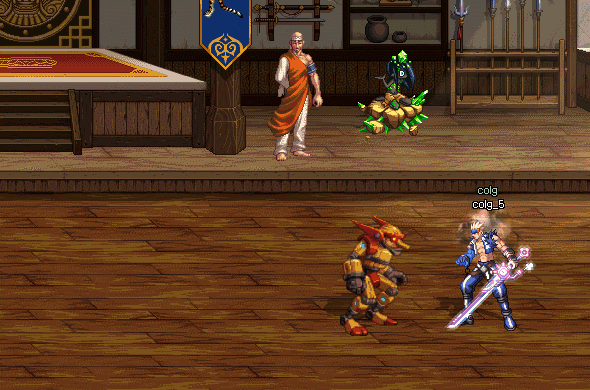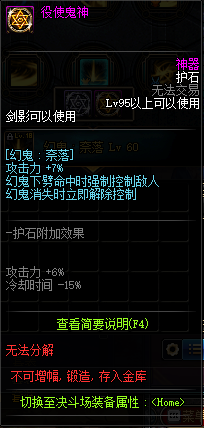100级工作服情况下，奈落等级可以达到23级

X% CD 17S

X%*1.13=1.13X% CD 14.5S

1.13X%/X%-1=13%